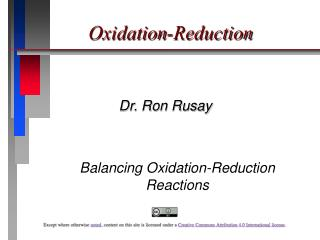DownloadDownload PresentationOxidation-Reduction

# Oxidation-Reduction

Download Presentation## Oxidation-Reduction

- - - - - - - - - - - - - - - - - - - - - - - - - - - E N D - - - - - - - - - - - - - - - - - - - - - - - - - - -
##### Presentation Transcript

1. Oxidation-Reduction Dr. Ron Rusay Balancing Oxidation-Reduction Reactions

2. Oxidation-Reduction • Oxidation is the loss of electrons. • Reduction is the gain of electrons. • The reactions occur together. One does not occur without the other. • The terms are used relative to the change in the oxidation state or oxidation number of the reactant(s).

3. Aqueous Reactions:Oxidation - Reduction • In the following reaction, identify what is being oxidized and what is being reduced. What is the total number of electrons involved in the process?

4. Oxidation Reduction Reactions

5. QUESTION In a redox reaction, oxidation and reduction must both occur. Which statement provides an accurate premise of redox chemistry? The substance that is oxidized must be the oxidizing agent. The substance that is oxidized must gain electrons. The substance that is oxidized must have a higher oxidation number afterwards. The substance that is oxidized must combine with oxygen.

6. QUESTION

7. QUESTION What is the oxidation number of chromium in ammonium dichromate? A) +3 B) +4 C) +5 D) +6

8. Zinc

9. Reactivity Tables (usually reducing) show relative reactivities: In the examples from the previous slide, the acid solution (H+) will react with anything below it in the Table but not above. Nickel and Zinc, ….but not Copper.

10. QUESTION • Select all redox reactions by looking for a change in oxidation number as reactants are converted to products. • I) Ca + 2 H2O → Ca(OH)2 + H2 • II) CaO + H2O → Ca(OH)2 • III) Ca(OH)2 + H3PO4→ Ca3(PO4)2 + H2O • IV) Cl2 + 2 KBr → Br2 + 2 KCl • A) I and II B) II and III C) I and IV D) III and IV

11. QUESTION

12. Number of electrons gained must equal the number of electrons lost. - 2 e- +2 e- Use oxidation numbers to determine what is oxidized and what is reduced. 0 +2 e- Cu 2+ Cu (s) 0 - 2 e- H2(g) 2 H + Refer to Balancing Oxidation-Reduction Reactions

13. QUESTION

14. Balancing Redox Equationsin acidic solutions 1) Determine the oxidation numbers of atoms in both reactants and products. 2) Identify and select out those which change oxidation number (“redox” atoms) into separate “half reactions”. 3) Balance the “redox” atoms and charges (electron gain and loss must equal!). 4) In acidic reactions balance oxygen with water then hydrogen from water with acid proton(s).

15. Balancing Redox Equationsin acidic solutions Fe+2(aq)+ Cr2O72-(aq) +H+(aq)  Fe3+(aq) + Cr3+(aq) + H2O(l) Fe 2+(aq)+ Cr2O72-(aq) +H+(aq)  Fe 3+(aq) + Cr 3+(aq) + H2O(l) ? Cr oxidation number? x =? Cr; 2x+7(-2) = -2; x = +6

16. Balancing Redox Equationsin acidic solutions Fe 2+(aq)  Fe 3+(aq) Cr2O72-(aq) +  Cr 3+(aq) Cr= (6+) -e - 2 6 e - 6 (Fe 2+(aq) -e -Fe3+(aq)) 6 Fe 2+(aq) 6 Fe3+(aq) + 6 e - Cr2O72-(aq) + 6 e -  2 Cr3+(aq)

17. Balancing Redox Equationsin acidic solutions 6 Fe2+(aq) 6 Fe3+(aq) + 6 e - Cr2O72-(aq) + 6 e -  2 Cr3+(aq) 6 Fe2+(aq)+ Cr2O72-(aq) + ? 2nd H+(aq)  6 Fe3+(aq) + 2 Cr3+(aq)+ ? 1st Oxygen H2O(l) Oxygen=7 2nd (Hydrogen)=14

18. Balancing Redox Equationsin acidic solutions Completely Balanced Equation: 6 Fe2+(aq)+ Cr2O72-(aq) + 14 H+(aq) 6 Fe3+(aq) + 2 Cr3+(aq)+ 7H2O(l)

19. QUESTION Dichromate ion in acidic medium converts ethanol, C2H5OH, to CO2 according to the unbalanced equation: Cr2O72−(aq) + C2H5OH(aq) → Cr3+(aq) + CO2(g) + H2O(l) The coefficient for H+ in the balanced equation using smallest integer coefficients is: A) 8 B) 10 C) 13 D) 16

20. Balancing Redox Equationsin basic solutions 1) Determine oxidation numbers of atoms in Reactants and Products 2) Identify and select out those which change oxidation number into separate “half reactions” 3) Balance redox atoms and charges (electron gain and loss must equal!) 4) In basic reactions balance the Oxygen with hydroxide then Hydrogen from hydroxide with water

21. Balancing Redox Equationsin basic solutions MnO2 (aq)+ ClO31-(aq) + OH 1-aq)  MnO41- (aq)+ Cl 1-(aq) + H2O(l) Mn4+ (MnO2)  Mn7+ (MnO4 )1- Cl+5(ClO3 )1-+ 6 e- Cl 1-

22. Balancing Redox Equationsin basic solutions Electronically Balanced Equation: 2 MnO2 (aq)+ ClO31-(aq) + 6e -  2 MnO4 1- + Cl 1- + 6 e-

23. Balancing Redox Equationsin basic solutions Completely Balanced Equation: 2 MnO2 (aq)+ ClO31-(aq) + 2OH 1- (aq) 2 MnO4 (aq)1- + Cl 1- (aq)+ 1 H2O (l) 9O in product

24. QUESTION Oxalate ion can be found in rhubarb and spinach (among other green leafy plants). The following unbalanced equation carried out in a basic solution, shows how MnO4– could be used to analyze samples for oxalate. MnO4– + C2O42– MnO2 + CO32– (basic solution) When properly balanced, how many OH– are present? 1 2 3 4